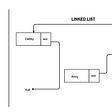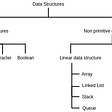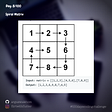# What is Big O notation??

According to wiki, Big O notation means “Big O notation is a mathematical notation that describes the limiting behavior of a function when the argument tends towards a particular value or infinity.”

Well, if you are like me, someone having zero knowledge about computer science or not majoring in computer, I believe you also don’t understand what it is talking about…

Basically, Big O notation is used to describe or to evaluate the performance of an algorithm/program, such as how fast it can run.
The performance can be evaluated in terms of space complexity(the memory it consumes) and time complexity. We will only be talking about time complexity because it’s more complicated.

When you create an algorithm and run it, it may finish the task instantly because the input is not large. You probably have only a few sets of data, let’s say ten or twenty sets. Your algorithm can process these ten or twenty sets of data instantly. However, what if you have a thousand or millions of sets of data. Your algorithm is sure to process this huge amount of data for a while. So, Big O notation is used to describe the performance of an algorithm when the input is very large.

However, how exactly is Big O notation used ? Let’s see common examples.

First, in Big O notation, we don’t actually describe the performance of an algorithm in terms of time because each line of code can be affected by many factors. For example, different computers have different equipment, thereby affecting the time a line of code takes to run. Even under different situations, the same line of code would take different time to run in the same computer. Therefore, an objective view of point is using how many operations or steps a line of code would take to describe the speed.

1. O(1) (pronounced as O of one):

The function above prints the first element of an input array. However, no matter how large the array is, it always only take one operation to finish. In this case, we use O(1) to indicate that the algorithm takes constant time to finsh the task.
Even if the algorithm looks like the following:

We still use O(1) to describe it because the algorithm always takes a constant number of operations to finish no matter how large the array is.

2. O(n) (pronounced as O of n):

The function above prints every single element in the input array, so how fast the function can run depends on the size of the input array. As a result, we use O(n) to indicate that the steps the function takes to finish increase linearly according to the size of the input. We say this algorithm runs in a linear time.

3. O(n²) (pronounced as O of n square)

It is a nested for loop. The outer loop iterates over each element in the input array, so it’s O(n). For each iteration, the inner loop also iterates over each element in the input array, so for the inner loop, it’s O(n) as well. As a result, we use O(n²) to describe the performance of the algorithm.

4. O(log n) (pronounced as O of log n)

Let’s say we have an array of numbers from 1 to 10. We’d like to find the number of 10. Generally, we can use for loop to iterate over each element to find the number. This is called linear search. Worst case scenario, 10 is the last one in the array, so we need to inspect each element. The runtime generally increases linearly according to the size of the array .

Alternatively, if the numbers are arranged in numerical order. We can use binary search to find the target number. First, we first look at the middle element to see if the middle number is less or greater than the target number. In our case, the middle number of 5 is less than the target number of 10. Then, the target number must be on the right partition. And then we repeat the same operations till we find the target number. With this binary search, we can significantly reduce our search.

5. O(2^n)

The instructor didn’t explain this very much. Basically, it is the opposite of O(log n). The runtime of an algorithm with O(2^n) increases exponentially.

I did some googling. Algorithms with running time O(2^n) are often recursive algorithms, and recursive algorithms basically are, a function calls itself inside itself.

One last thing, an algorithm usually contains many code, so it may have different Big Os. However, the Big Os are generally simplified, and only the most critical one (the most time-consuming) is used.

The graph below is a brief summary of the big O notations described above.

If there’s anything incorrect, please let me know.

--

--

--

Love podcasts or audiobooks? Learn on the go with our new app.

## Chit chat with a lady techie, KBwomenintech face of the month## Terratest, hands-on## Understanding the Kubernetes manifest## Text Recognition in Flutter Using Firebase’s ML Kit## Keep Trying and Never Give Up## Data Structure — Linked List## Explore Data Structure and Algorithm Part-2## Spiral Matrix — Problem solution